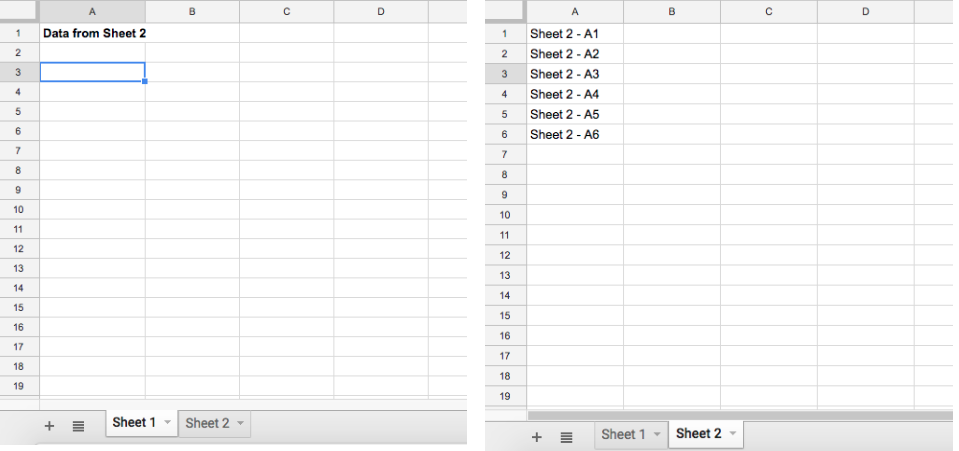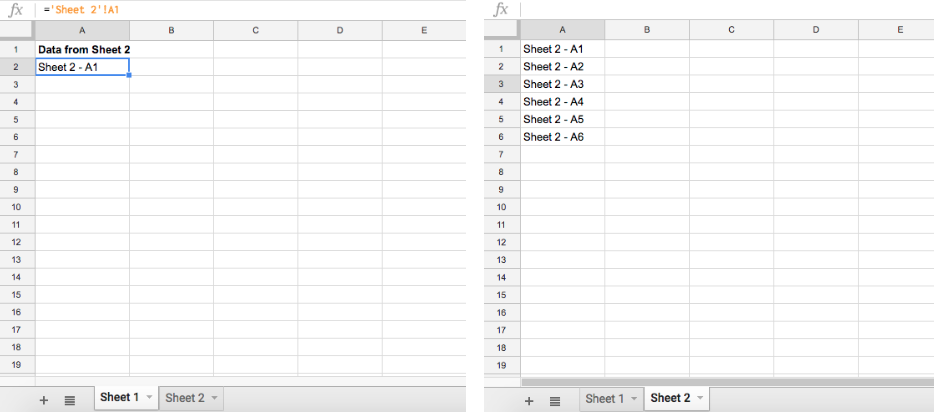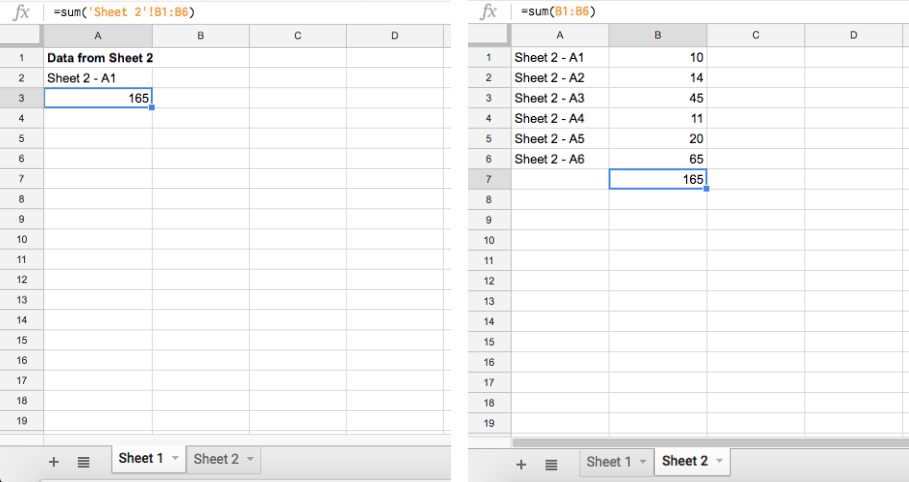Get instant live expert help with Excel or Google Sheets“My Excelchat expert helped me in less than 20 minutes, saving me what would have been 5 hours of work!”

#### Post your problem and you’ll get Expert help in seconds.

Your message must be at least 40 characters
Our professional Expert are available now. Your privacy is guaranteed.

# How to Pull Data from Another Sheet in Google Sheets

Pulling Data from another sheet in Google Sheets is a very simple process. This tutorial will walk through the steps to pull data from other sheets in Google Sheets

## Setting up the Data

For this Example, we will use two Sheets. Sheet 1- cell A2 will be the formula cell. Sheet 2 will be the reference Sheet where we will pull the data from. In Column A of Sheet 2, enter some test data to reference in our formulaFigure 1 – Sheets 1 an 2 Data Setup

## Applying the formula Single Cell

Now that we have our data setup, the next step is to enter our formula in cell A1 of Sheet 1

Formula Syntax: `=‘Sheet Name’!Cell`

• In cell A1 of Sheet 1, enter this formula =’Sheet2’!A1Figure 2 – Applying the Formula in a Single Cell

We can see in this example that by entering the referencing  Sheets name in this format ‘Sheet Name!’, the formula pulled the data associated with Sheet 2 into Sheet 1.

## Applying the formula to a Range

Now that we know how to apply the formula to a single cell, we can use the same concept to apply to a range of data. Not only will we apply this formula into a range of data, but we will illustrate how we can nest this formula inside others in order to reference other sheets in any formula

• In Column B of Sheet 2, enter some numeric data
• In cell B7 of Sheet 2, enter this formula =sum(B1:B6)
• In cell A3 of Sheet 1, enter this formula = sum(‘Sheet 2’!B1:B6)Figure 3 – Applying the Formula to a Range

We can see in this example the formula in Sheet 1, cell A2 sums the data in range B1:B6 of Sheet 2 to match the sum result in Sheet 2, cell B7. This same concept can be applied for any Excel formula

Most of the time, the problem you will need to solve will be more complex than a simple application of a formula or function. If you want to save hours of research and frustration, try our live Excelchat service! Our Excel Experts are available 24/7 to answer any Excel question you may have. We guarantee a connection within 30 seconds and a customized solution within 20 minutes.

Are you still looking for help with the VLOOKUP function? View our comprehensive round-up of VLOOKUP function tutorials here.